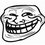# One thing I learned when doing Brilliant problems

If it says "put $x$ as the answer if ..." in the clarification, then $x$ is not the answer.Note by Daniel Wang
7 years ago

This discussion board is a place to discuss our Daily Challenges and the math and science related to those challenges. Explanations are more than just a solution — they should explain the steps and thinking strategies that you used to obtain the solution. Comments should further the discussion of math and science.

When posting on Brilliant:

• Use the emojis to react to an explanation, whether you're congratulating a job well done , or just really confused .
• Ask specific questions about the challenge or the steps in somebody's explanation. Well-posed questions can add a lot to the discussion, but posting "I don't understand!" doesn't help anyone.
• Try to contribute something new to the discussion, whether it is an extension, generalization or other idea related to the challenge.

MarkdownAppears as
*italics* or _italics_ italics
**bold** or __bold__ bold
- bulleted- list
• bulleted
• list
1. numbered2. list
1. numbered
2. list
Note: you must add a full line of space before and after lists for them to show up correctly
paragraph 1paragraph 2

paragraph 1

paragraph 2

[example link](https://brilliant.org)example link
> This is a quote
This is a quote
    # I indented these lines
# 4 spaces, and now they show
# up as a code block.

print "hello world"
# I indented these lines
# 4 spaces, and now they show
# up as a code block.

print "hello world"
MathAppears as
Remember to wrap math in $$ ... $$ or $ ... $ to ensure proper formatting.
2 \times 3 $2 \times 3$
2^{34} $2^{34}$
a_{i-1} $a_{i-1}$
\frac{2}{3} $\frac{2}{3}$
\sqrt{2} $\sqrt{2}$
\sum_{i=1}^3 $\sum_{i=1}^3$
\sin \theta $\sin \theta$
\boxed{123} $\boxed{123}$

Sort by:

Your statement is not necessarily true.

Staff - 7 years ago

Next week, be sure to try $x$ for all problems with such a clarification :)

- 7 years ago

thats a good idea

- 7 years ago

I actually learn something a little bit different. For example,

• "The answer can be expressed as $\dfrac{a}{b}$, where $a,b$ are coprime positive integers. Find $a+b$." usually means $b \neq 1$, otherwise they will ask for the answer straight away.
• "Find the last three digits of the answer." usually means the answer is greater than or equal to $1000$, otherwise they will ask for the answer straight away.

Note all "usually"s appearing there, so don't blame me for blindly following the above.

- 7 years ago

Likewise, not necessarily true. I do try and avoid allowing you to make such generalizations. The assumption that the answer must be an integer from 0 to 999 is introduced for simplicity in explanation. We might remove that condition in future, and use the Physics style of "real numbers" instead.

If a value is 'clearly' in the form of a fraction (e.g. expected value, lots of division going on, etc) I often ask in terms of a fraction, even if the answer turns out to be an integer. Though, to be fair, this is much rarer.

If a value is 'potentially' huge (e.g. find the sum of all numbers which satisfy this condition), I often ask for the last three digits. I've received numerous disputes saying that "but the answer must be more than 1000, so you are wrong".

Staff - 7 years ago

Well, I rarely see problems that disprove the above claims, and I do claim "usually", so my claims still stand. But I've never deduced in that way anyway.

A related note, a problem just last week: "Find the sum of all $a$ satisfying the condition." I got one possible value of $a$ that was a fraction; everything else were integers. I had the strong urge to dismiss that fractional value by "if that fractional value is a possible value of $a$, then the answer of this question will not be an integer".

- 7 years ago

Actually for the second one, it quite often is less than $1000$, but simply is there to not have you discount the possibility that it is greater than $1000$ (which can, conceptually, be a huge indicator in problems of the scope you're dealing with)

- 7 years ago

I know you said 'usually' but here is a counterexample to the second point.

<https://brilliant.org/assessment/s/number-theory/5045346/>

- 7 years ago

Okay, I notice the "IF", but why "x" is not the answer?

well.. it could've said put x if x is greater than or equal to 0 else put x + 1000.

- 7 years ago

guys, pls anyone tell me! how do I create a challenge? thanks, john

- 7 years ago

You can't now that they removed the option to submit problems.

- 7 years ago

I know i really liked that :(

- 7 years ago

They want x as the answer in the first place, so why is it NOT the answer? I don't get you. EDIT: Assuming the 'if...' is proven true in the question.

- 7 years ago

Assuming the 'if...' is proven true in the question.

It usually isn't true.

- 7 years ago